## The Set of CM Elliptic Curves with Given CM

Definition 3.6 (Fractional Ideal)   A fractional ideal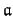of a number field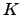is an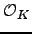-submodule ofthat is isomorphic to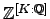as an abelian group. In particular,is nonzero.

Ifis a fractional ideal, the inverse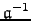of, which is the set of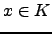such that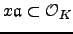, is also a fractional ideal. Moreover,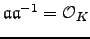.

Fix a quadratic imaginary field. Let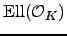be the set of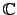-isomorphism classes of elliptic curves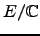with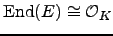. By the above results we may also viewas the set of lattices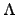with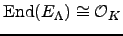.

Ifis a fractionalideal, then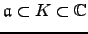is a lattice in. For the elliptic curve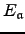we have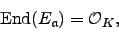becauseis an-module by definition. Since rescaling a lattice produces an isomorphic elliptic curve, for any nonzero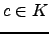the fractional idealsand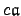define the same elements of.

The class group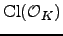is the group of fractional ideals modulo principal fractional ideals. Ifis a fractionalideal, denote byits ideal class in the class groupof. We have a natural map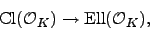which sendsto.

Theorem 3.7   Fix a quadratic imaginary field, and letbe a lattice insuch that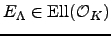. Letand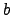be nonzero fractional-ideals. Then
1.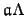is a lattice in,
2. We have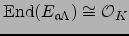.
3. We have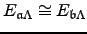if and only if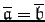.
Thus there is a well-defined action ofongiven by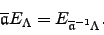Theorem 3.8   The action ofonis simply transitive.

Example 3.9   Let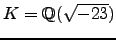. Then the class number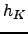is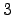. An elliptic curve with CM byis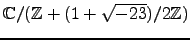, and one can obtain the other two elements ofby multiplying the lattice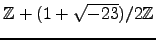by two representative ideal classes for.

William 2007-05-25